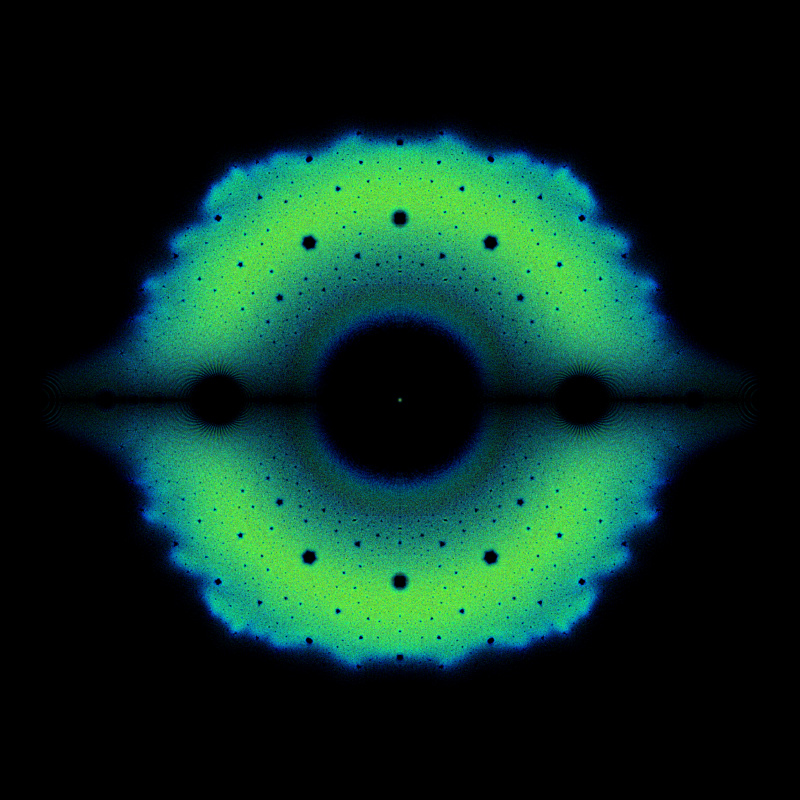# Bohemian MatricesA density plot in the complex plane of the Bohemian eigenvalues of a sample of 20 million 8x8 $(p=7)$ doubly companion matrices: $$\begin{bmatrix} -\alpha_1 & -\alpha_2 & \cdots & -\alpha_p & -\alpha_{p+1} - \beta_{p+1}\\ 1 & 0 & \cdots & 0 & -\beta_p\\ 0 & 1 & \cdots & 0 & -\beta_{p-1}\\ \vdots & \vdots & \ddots & \vdots & \vdots\\ 0 & 0 & \cdots & 1 & -\beta_1 \end{bmatrix}$$ with $\alpha_k \in \{-1, 0, 1\}$, and $\beta_k \in \{-1, 0, 1\}$. Color represents the eigenvalue density and the plot is viewed on [-2.2-2.2i, 2.2+2.2i].